The structural frame
The construction
The reinforcement I
The reinforcement II
Quantity/Cost estimation
Detailing drawings
Introduction >Wind and Seismic Forces >
Structural model and Analysis
Slabs
Seismic behavour of frames
Appendix A
Appendix B
Appendix C
Appendix D
Introduction >
Modelling slabsMaterials
To be continued >
Introduction

 1 2 3 4 5 6

 1 2 3 4 5 6

 1 2 3 4 5 6

 1 2 3 4 5 6

 1 2 3 4 5 6

 1 2 3 4 5 6

 1 2 3 4 5 6

max M1,

max M4,

max M5,

min M2,

min M3,

min M6

max M2,

max M3,

max M6,

min M1,

min M4,

min M5

max |M1-2|

max |M5-6|

min |M3-4|

max |M1-3|,

min |M2-4|

max |M2-4|,

min |M1-3|

max |M3-5|,

min |M4-6|

max |M4-6|,

min |M3-5|

The minimum load applied on a slab is equal to g whereas the maximum load is equal to p=(γg-1)×gi + γq×qi. Τhe general problem concerns the way that the slabs should be loaded for developing maximum stresses.

This is a complicated issue since, even for a simple case of six slabs arranged in a grid seven unfavourable loadings are required, as illustrated in the following figure. The analysis of such a simple example via tables can be performed only for equally grid axes.

The problem becomes even more complicated when slabs are not arranged in a grid.

General and accurate analysis can only be achieved by means of the the finite element method. However, the resources for such an analysis require sophisticated algorithms and modern computers. The related software performs parallel processing of such algorithms on all available cores of a personal computer providing solutions to such complicated problems within seconds.

The unfavourable loadings on the three previously resolved examples will be examined next.

## 1st example: Shear forces envelopes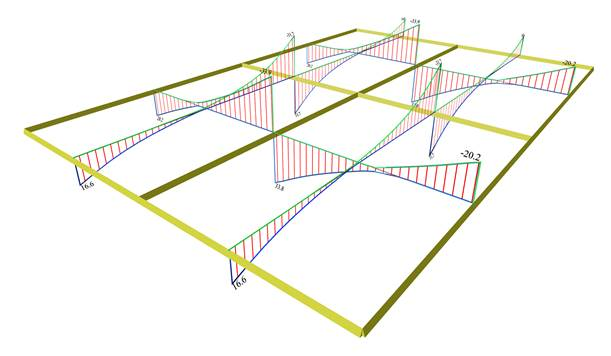project <Β_422-1>

The most unfavourable shear forces are equal to:

x direction: Vxr= 20.2 (18.8), Vx,erm=-33.8 (-33.3) [kN]

y direction: Vyr=16.6 (15.1), Vy,erm=-31.7 (-29.0) [kN]

Note that although the live load has a relatively high value, the differences between the shear forces do not exceed 8%.

## 1st example: Bending moment envelopes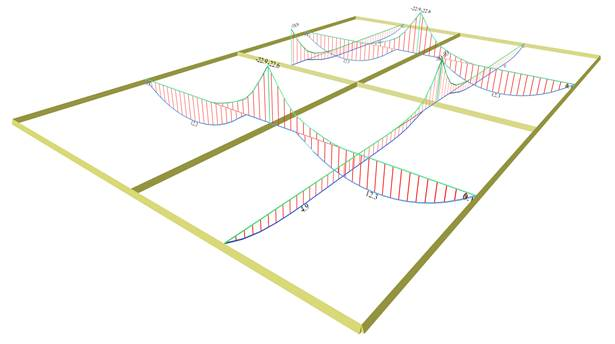The most unfavourable bending moments are equal to:

x direction: Mx=12.3 (10.8), Mx,erm=-22.8 (-22.0) [kNm]

y direction: My=4.9   (4.1),   My,erm=-18.8 (-16.3) [kNm]

Note that although the live load has a relatively high value , the differences between the bending moments are small and less than15%.

## 1st example: Deflection envelopes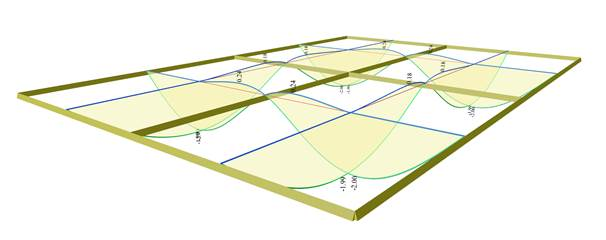The largest slab deflection is equal to y=-2.00 (-1.53) mm and y=+0.24 mm. It is concluded that the slab is lifted, which is in contrast with the response of the global loading case, due to sym-metry of course.
Notice that the deflections are as high as 30% while opposite sign deformations also arise (blue lines).

## 2nd example: Shear forces envelopes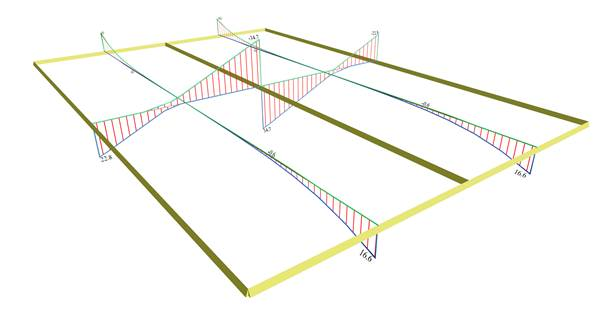project <Β_422-2>

The most unfavourable shear forces are equal to:

x direction: Vxr=22.8 (20.6), Vx,erm=-34.7 (-34.7) [kN]

y direction: Vyr=16.6 (15.3) [kN]

Notice that although the live load has a relatively high value , the shear forces do not differ more than 10%. Of course , this is due to the fact that only 2 spans exist and the unfavourable shear forces at the supports result from the global loading 1.35g+1.50q. The differences would be even higher in multiple spans.

## 2nd example: Bending moment envelopes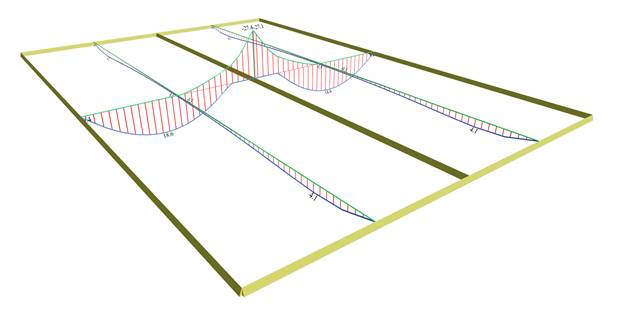The most unfavourable bending moments are equal to:

x direction: Mx=18.6 (15.5), Mx,erm=-27.4 (-27.4) [kNm]

y direction: My=4.1 (3.6) [kNm]

Note that although the live load has a relatively high valuethe differences between the moments are small and less than 20%.

## 2nd example: Deflection envelopes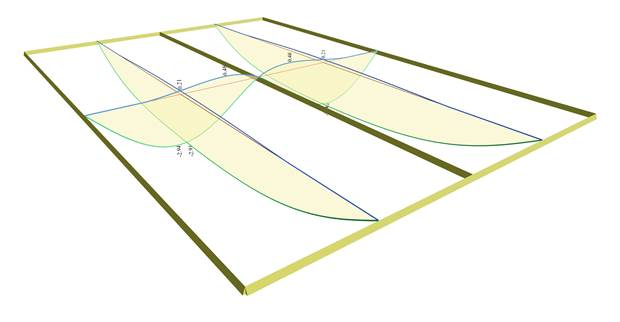The most unfavourable slab deflection is equal to y=-2.94 mm (-2.10 mm) and y=+0.48, i.e. the slab is lifted in this case as well, in contrast with the response of global loading case. Notice that the deflections are as high as 40% while opposite sign deformations also arise (blue lines).

## 3rd example: Region of three-edge- supported slabs Shear forces envelopes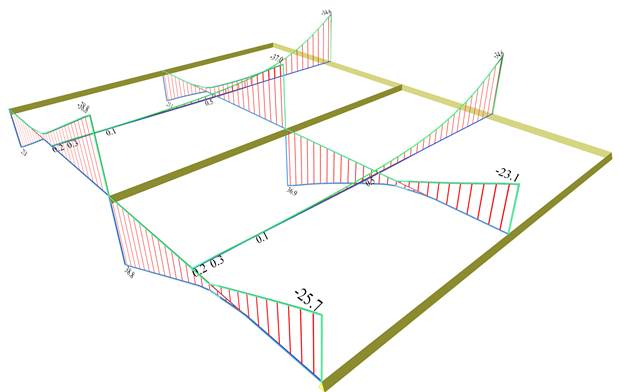project <Β_422-3>

The most unfavourable shear forces are equal to:

Middle cross-section in x: Vxr=23.1 (21.1), Vx,erm=-37.0 (-36.4) [kN]

End cross-section in x: Vxr=25.8 (23.1), Vx,erm=-38.8 (-38.3) [kN]

y direction: Vy,erm=-34.6 (-31.3) [kN]

Note that although the live load has a relatively high value, the differences between the shear forces do not exceed 9%.

## 3rd example: Region of three-edge- supported slabs Bending moment envelopes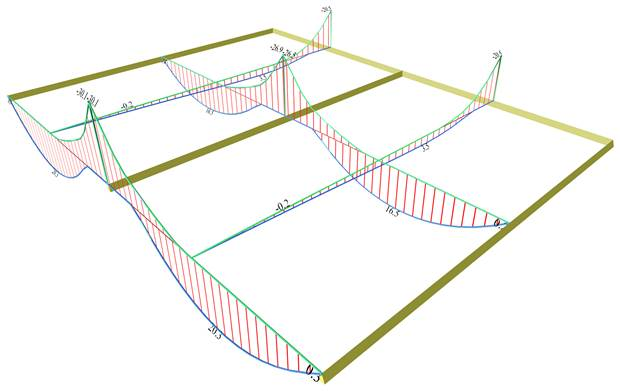The most unfavourable bending moments are equal to:

Middle cross-section in x: Μx=16.5 (13.8), Mx,erm=-26.7 (-26.6) [kNm]

End cross-section in x: Mx=20.3 (16.9), Mx,erm=-29.9 (-29.9) [kNm]

y direction: My=3.5 (2.8), My,erm=-20.5 (-17.4) [kNm]

Notice that although the live load has a relatively high value, the differences between the bending moments are small

.

## 3rd example: Region of three-edge- supported slabs Deflection envelopes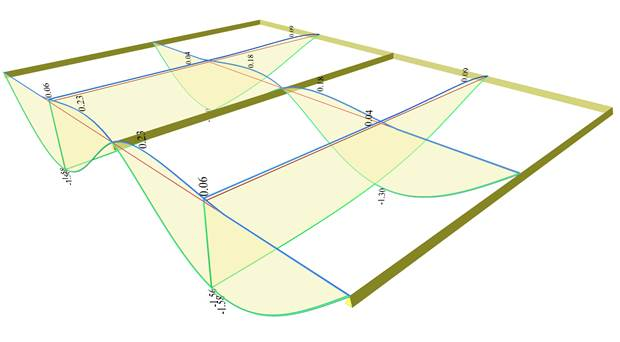Maximum deflections of y=-1.58 (-1.14 ) mm occur at the free edges of the three-edge-supported slabs. At the same edges opposite sign deformation of y=0.23 mm is also developed.
In the middle cross-section of the slabs the extreme deflections are y=-1.30(- 0.94) mm and y=0.18 mm respectively.
Note that the deflections are quite higher reaching up to 40% and, at the same time, opposite sign deformations also arise (blue lines).

## 3rd example: Cantilever region Shear forces envelopes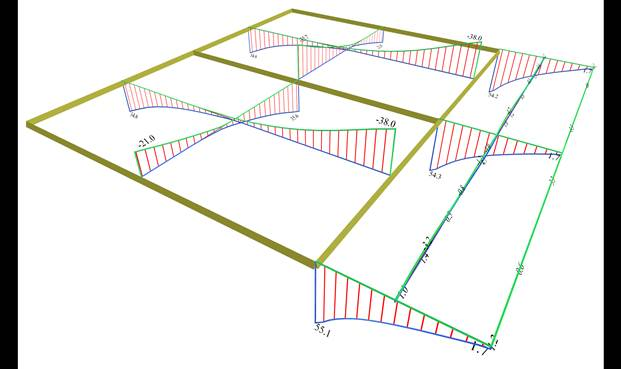The most unfavourable shear forces are equal to:

Two-way in x: Vxr=20.0 (18.6), Vx,erm=-35.7 (-33.6) [kN]

Cantilever in x: small shear forces

Two-way in y: Vyr=34.6 (31.4), Vy,erm=-38.0 (-35.9) [kN]

Cantilever in y: Vy,erm=54.3 (46.9) [kN]

Notice that although the live load has a relatively high value, the differences between the shear forces do not exceed 6% except for the peak of the cantilever middle support reaching 15%

.

## 3rd example: Cantilever region Bending moment envelopes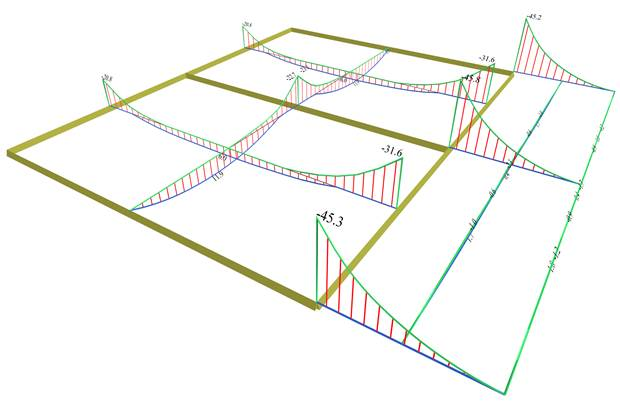The most unfavourable bending moments are equal to:

Two-way in x: Μx=11.9 (8.0), Mx,erm=-22.8 (-19.9) [kNm]

Cantilever in x: small moments

Two-way in y: Μy=6.0 (4.9), My,erm,1=-20.5 (-17.4) and My,erm,2=-31.6  (-30.6) [kNm]

Cantilever in y: extended My,erm =-31.6 (-30.6), peak -45.8 (-41.3) [kNm]

The differences between the unfavourable bending moments and the bending moments due to global loading, range from 15 to 50%

.

## 3rd example: Cantilever region Deflection envelopes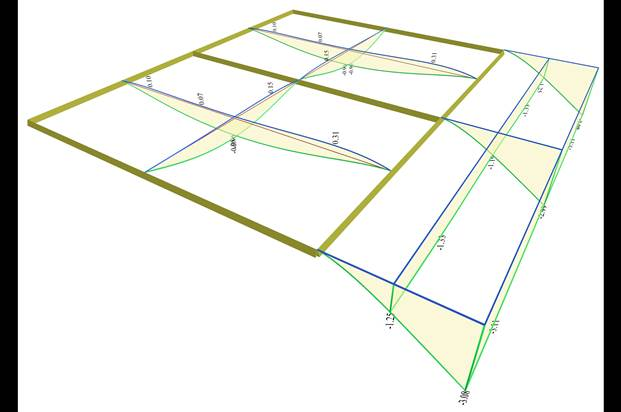Maximum deflection of y=-0.96 (-0.64) mm and opposite sign deflection of y=0.31 (0.09) mm occur in two-way slabs.
In cantilever slab the maximum deflection is y=-3.11 (-2.81) mm.
The slightly fluctuating deformations of the cantilever longitudinal free edge, are due to the fluc-tuations of the two-way slabs deformations behind the cantilever.

## Clarifications: (1) The “BUILDING” module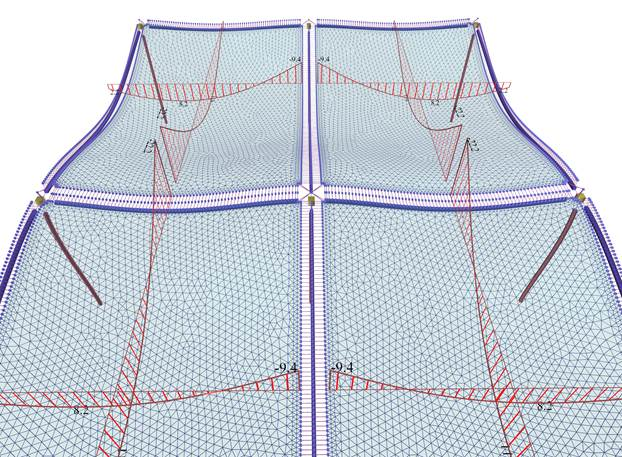In module ‘BUILDING’, the interaction of the slabs with the structure is taken into account resulting from point to point more or less favourable stresses compared to the module ‘SLABS’.

## Clarifications: (2) Multistorey buildings and envelopes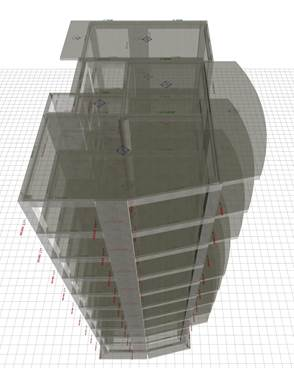The actual structure in 3D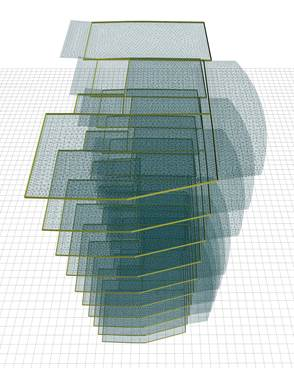The structure’s model in 3D
For the slab analysis, the unfavourable loadings  are created per floor, i.e. loading of any floor slab has no effect on slabs of other floors. In “SLABS” module this effect is obvious while in “BUILDING” module is rather insignificant.